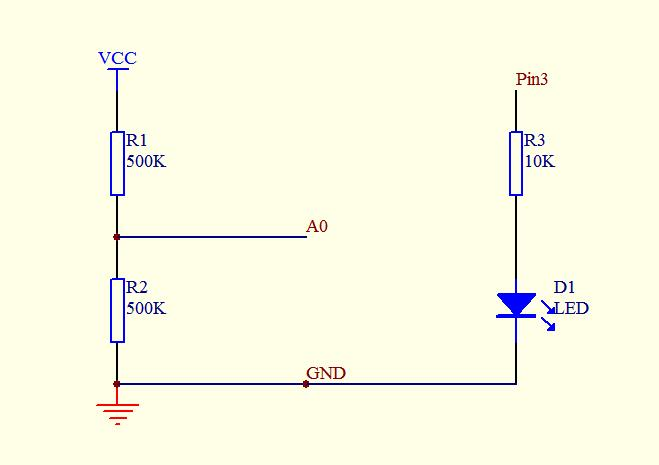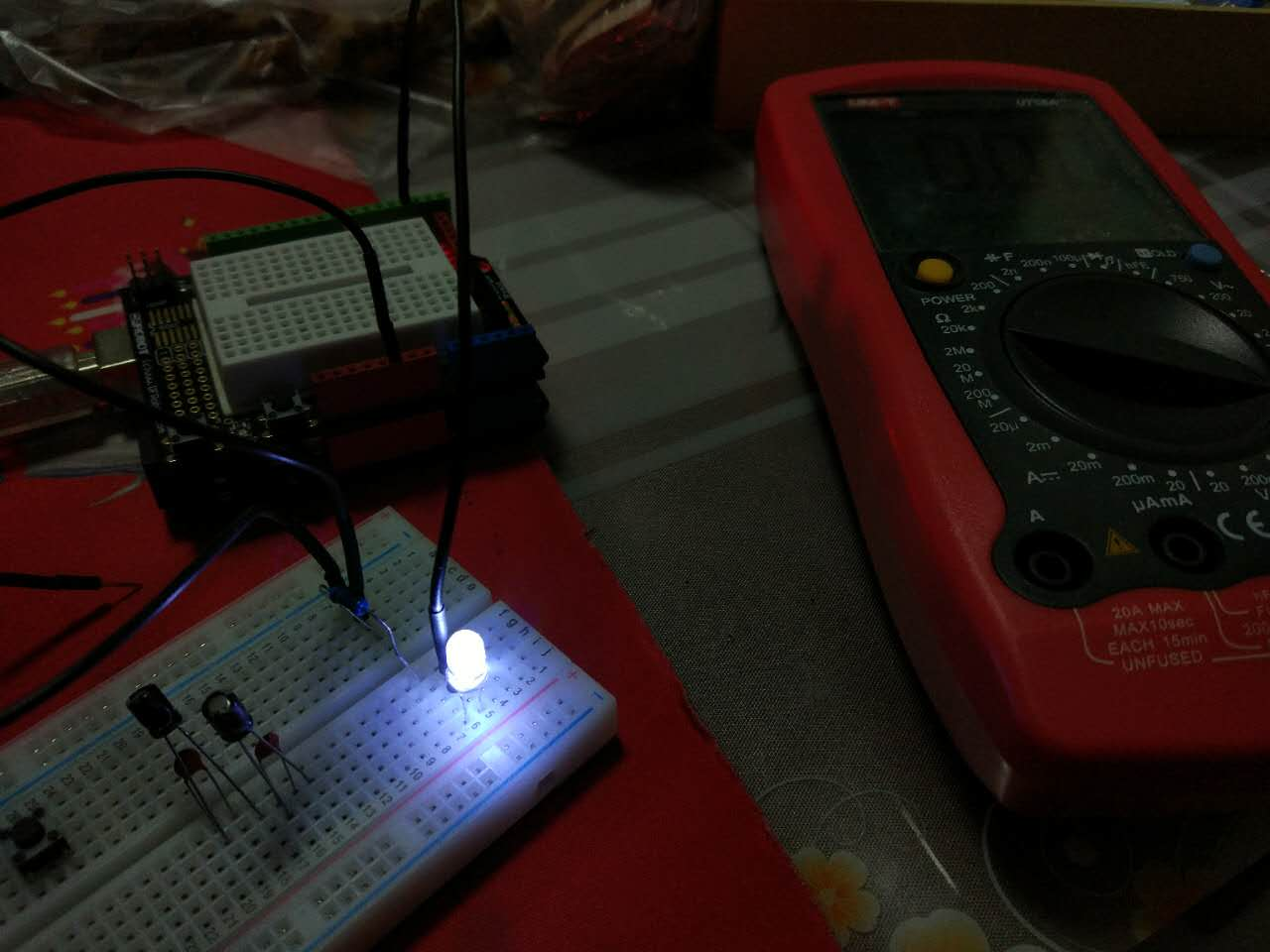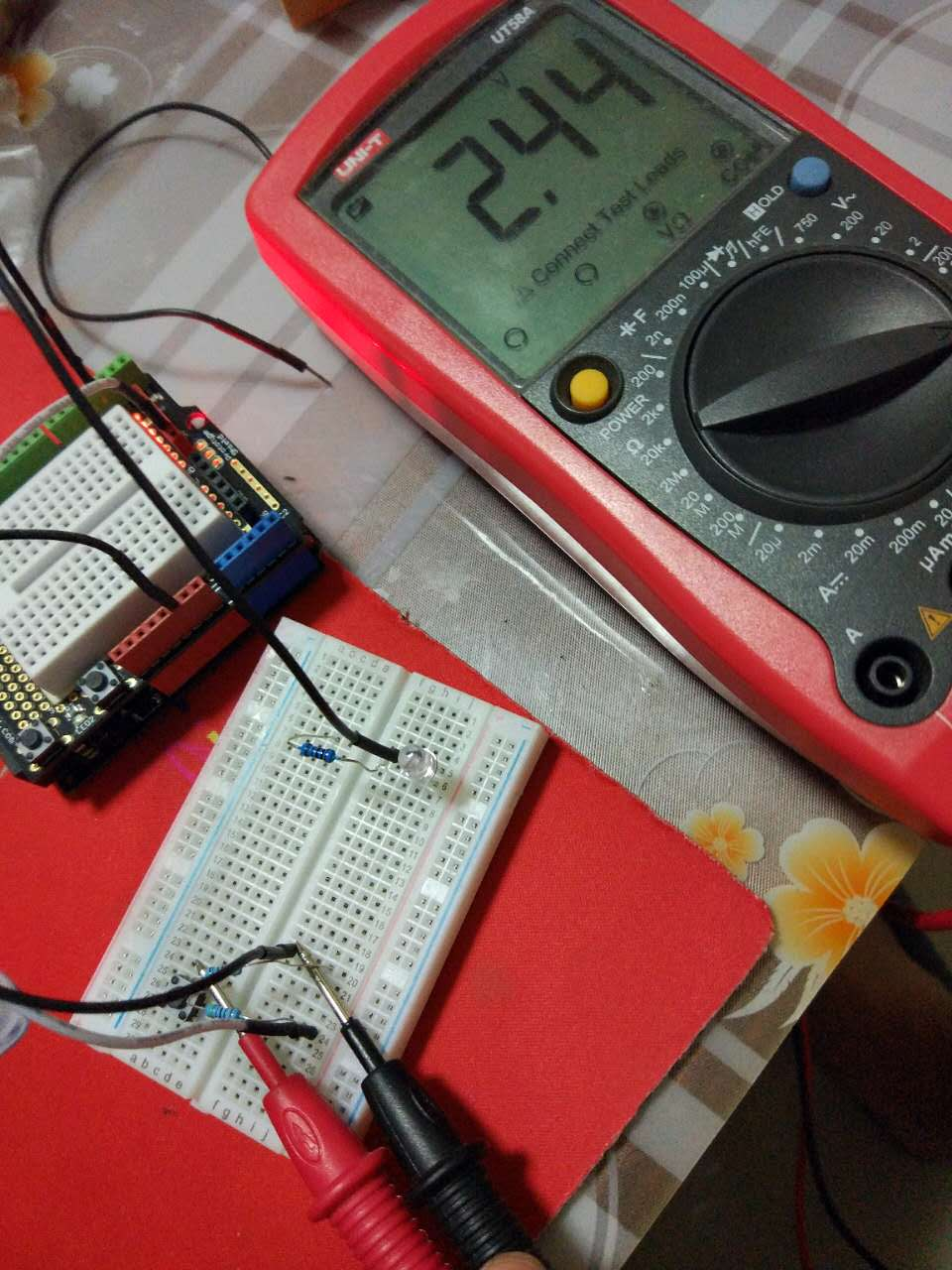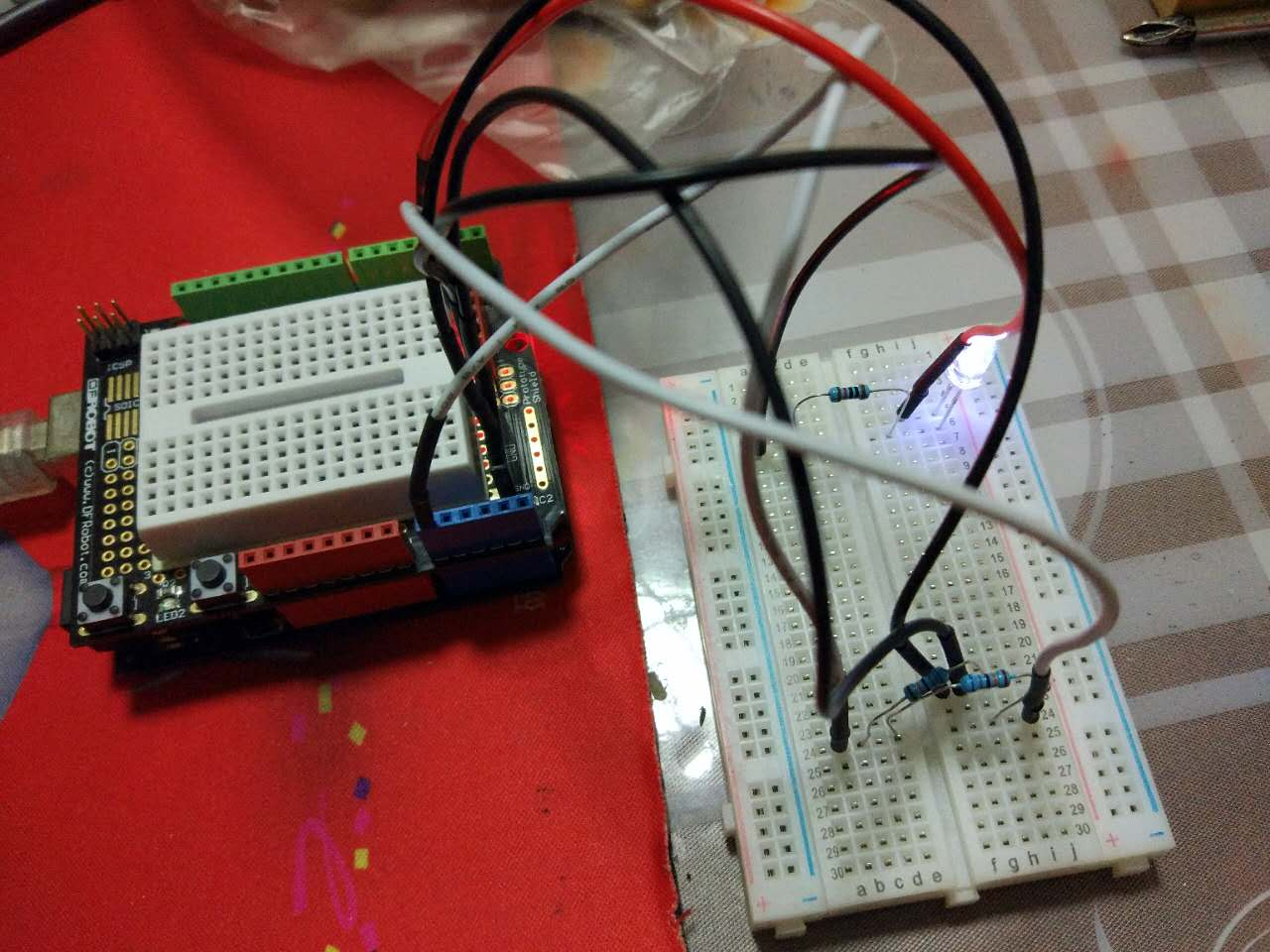# 智能小车21：电压检测模块设计```float tVoltage=0;//ao读取电压值
float tCurrent; //电流
float tResistance=500000;//电阻
int tVcc=5;//电压换算单位,最大5v
int limitVoltage=3;//电压在这个数以下就报警
int time=0;//计数
int lightFlagPin=3;//电灯开头引脚
void setup() {
pinMode(lightFlagPin,OUTPUT);
digitalWrite(lightFlagPin,LOW);
Serial.begin(9600);  //定义串口波特率为9600
}

void loop() {
time=time+1;
tVoltage=tVoltage/1023*tVcc;  //换算为电压值
if(tVoltage<limitVoltage){
digitalWrite(lightFlagPin,HIGH);
}else{
digitalWrite(lightFlagPin,LOW);
}
tCurrent=tVoltage/tResistance;
delay(3000);        // 3秒刷新一次
}```

### 相关阅读↓ 广告开始-头部带绿为生活 ↓
↑ 广告结束-尾部支持多点击 ↑